A color gradient background will change the color from a start color to a finish color in even steps (the number of steps depends on the size of the graph). The direction of the gradient is controlled by specifying a style parameter for the gradient. The signature for the gradient background method is

1. `Graph::SetBackgroundGradient(\$aFrom='navy',\$aTo='silver',\$aGradType=2,\$aStyle=BGRAD_FRAME)`

The last style par mater specified where in the graph the gradient style should be applied and the different options are

• `BGRAD_FRAME`, The entire graph frame will be filled

• `BGRAD_MARGIN`, Only the margin area (the graph area outside the plot area) will be filled

• `GRAD_PLOT`, Only the plot area will be filled

This is illustrated in Figure 14.93

Figure 14.93. What area of the graph the gradient should affect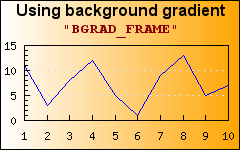`BGRAD_FRAME`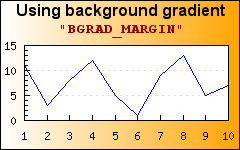`BGRAD_MARGIN`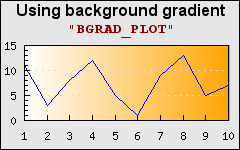`BGRAD_PLOT`

The different types of gradient fills are shown in Figure 14.94 where the gradient from color is "`white`" and the to color is "`orange`"

Figure 14.94. Different types of gradient fills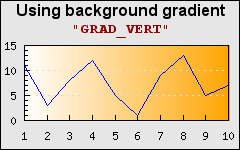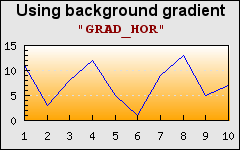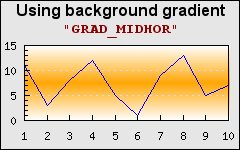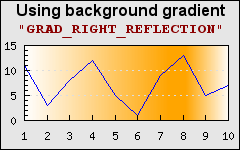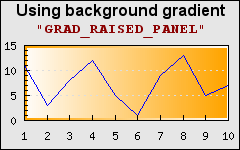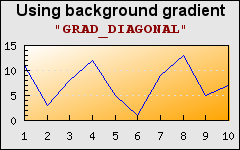### 14.16.1. Generating gradient background off-line

A drawback of using gradient backgrounds is that they are processing intensive and since they are generated each time the graph is generated it can take substantive amount of time for large graphs. To help with this the library offers a small (very simple) utility that can be tun to create gradient images off line. These images can later on be used as (static) background images. In the distribution there is a small utility in the "`Examples/`" directory called "`mkgrad.php`". Pointing the browser to this script will show a basic form as shown in Figure 14.95. The script will allow the creation of a gradient images using any of the predefined colors and gradient types.